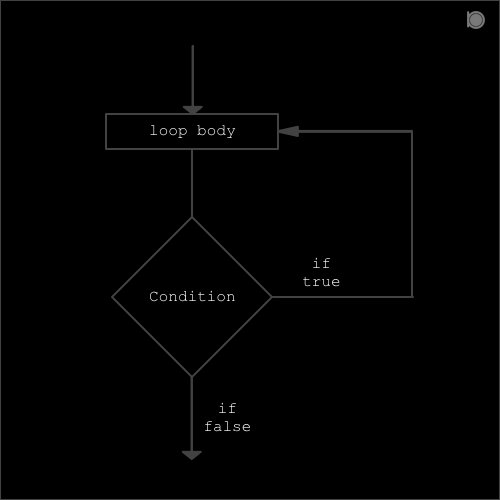do..while loop is similar to while loop except, in do while loop the loop body is executed at least one time even if the condition is false but in while loop the loop body is never executed if the condition is false.In the do..while loop the loop body is executed for first time and then the condition is checked and if the condition is true then again the loop body is executed but if the condition is false then the loop body is not executed.

##### Syntax for while loop in C:
``````do
{
statements;
}
while(condition or expression);
``````

Here condition or expression can be true or false.

### Program example of do while loop

``````//An example of do while loop
#include<stdio.h>

int main()
{
int x = 1;
do
{
printf("%d\n",x);
x++;
}
while (x<10)
return 0;
}
``````

### output

``````1
2
3
4
5
6
7
8
9
``````

Here in above example we have taken a variable x = 1. Now when the control reaches to do..while loop then first of all the loop body is executed. This means it will print output as 1 and then post increment the value of x by 1 which means the value of x will be 2.

Now after the execution of the loop body the condition is checked, here our condition is x<10 and our condition is true because 2 < 10. So the loop will run again and print output 2. This will repeat till the value of x becomes 9. And if the value of x becomes 10 then the condition becomes false and the control will exit out of the do..while loop and now the control is transferred to the statements right next to the do..while loop.

### Lets take another example

``````//An example of do..while loop when the condition is false initially
#include<stdio.h>

int main()
{
int x = 1;

do
{
printf("%d\n",x);
x++;
}
while(x<0);
return 0;
}

``````

### output

``````1
``````

In the above example we saw that our output is 1 even if our condition is false (1 is not less than 0). This is because in do..while loop the loop body is executed at least one time even if the condition is false and then the condition is checked.